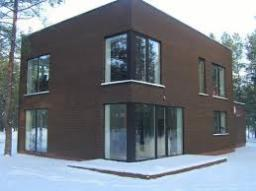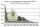# Real estate

Residential house has three entrances numbered by odd numbers arithmetic progression. The sum of the two numbers on the corner entrances is 50. Calculate the highest of this three numbers.

Result

m =  27

#### Solution:

m+(m-4)=50

2m = 54

m = 27

Calculated by our simple equation calculator.Our examples were largely sent or created by pupils and students themselves. Therefore, we would be pleased if you could send us any errors you found, spelling mistakes, or rephasing the example. Thank you!

Leave us a comment of this math problem and its solution (i.e. if it is still somewhat unclear...):Be the first to comment!#### Following knowledge from mathematics are needed to solve this word math problem:

Do you solve Diofant problems and looking for a calculator of Diofant integer equations? Do you have a linear equation or system of equations and looking for its solution? Or do you have quadratic equation?

## Next similar math problems:

1. GardenArea of a square garden is 6/4 of triangle garden with sides 56 m, 35 m, and 35 m. How many meters of fencing need to fence a square garden?
2. Forestry workersIn the forest is employed 56 laborers planting trees in nurseries. For 8 hour work day would end job in 37 days. After 16 days, 9 laborers go forth? How many days are needed to complete planting trees in nurseries by others, if they will work 10 hours a d
3. CoffeeIn stock are three kinds of branded coffee prices: I. kind......205 Kc/kg II. kind......274 Kc/kg III. kind.....168 Kc/kg Mixing these three species in the ratio 8:5:6 create a mixture. What will be the price of 100 grams of this mixture?
4. BonusGross wage was 527 EUR including 16% bonus. How many EUR were bonuses?
5. Motion problemFrom Levíc to Košíc go car at speed 81 km/h. From Košíc to Levíc go another car at speed 69 km/h. How many minutes before the meeting will be cars 27 km away?
6. TroopsThe route is long 147 km and the first-day first regiment went at an average speed of 12 km/h and journey back 21 km/h. The second day went second regiment the same route at an average speed of 22 km/h there and back. Which regiment will take route longe
7. ExcavationMr. Billy calculated that excavation for a water connection dig for 12 days. His friend would take 10 days. Billy worked 3 days alone. Then his friend came to help and started on the other end. On what day since the beginning of excavation they met?
8. DiophantusWe know little about this Greek mathematician from Alexandria, except that he lived around 3rd century A.D. Thanks to an admirer of his, who described his life by means of an algebraic riddle, we know at least something about his life. Diophantus's youth l
9. MotionIf you go at speed 3.7 km/h, you come to the station 42 minutes after leaving the train. If you go by bike to the station at speed 27 km/h, you come to the station 56 minutes before its departure. How far is the train station?
10. StoreOne meter of the textile was discounted by 2 USD. Now 9 m of textile cost as before 8 m. Calculate the old and new price of 1 m of the textile.
11. HandsThe clock shows 12 hours. After how many minutes will angle between the hour and minute hand 90°? Consider the continuous movement of both hands hours.
12. Rectangular cuboidThe rectangular cuboid has a surface area 5334 cm2, its dimensions are in the ratio 2:4:5. Find the volume of this rectangular cuboid.
13. Motion2Cyclist started out of town at 19 km/h. After 0.7 hours car started behind him in the same direction and caught up with him for 23 minutes. How fast and how long went car from the city to caught cyclist?
14. RootThe root of the equation ? is: ?
15. Bus vs. trainBus started from point A 10 minutes before the train started from the same place. The bus went an average speed of 49 km/h, train 77 km/h. To point B train and bus arrived simultaneously. Calculate time of train journey, if train and bus travelled the.
16. Right triangle AlefThe obvod of a right triangle is 84 cm, the hypotenuse is 37 cm long. Determine the lengths of the legs.
17. InflationWhat is better for people (employees)? ?